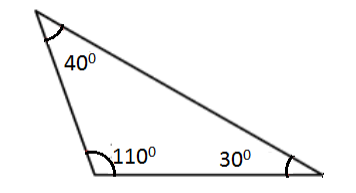QuestionAnswers

# Identify the name of the triangle.Verified
Hint: An acute triangle has all angles measuring less than ${90^ \circ }$. An oblique triangle is any triangle that is not a right triangle. It may be acute, obtuse, equiangular, scalene, isosceles, or equilateral, but not a right triangle.
Complete step by step solution: The angles in a scalene triangle are also of different measures. A scalene triangle has three sides of different lengths and no congruent sides. An isosceles triangle has two congruent sides. The two sides in the isosceles triangle are the same. An equilateral triangle has three congruent sides. A right-angle measure exactly ${90^ \circ }$. An equiangular triangle has three congruent angles. Each angle has a measure of ${60^ \circ }$. An obtuse triangle has one angle measuring more than ${90^ \circ }$ but less than ${180^ \circ }$ (an obtuse angle). It is not possible to draw a triangle with more than one obtuse angle. An oblique triangle is any triangle that is not a right triangle. It may be acute, obtuse, equiangular, scalene, isosceles, or equilateral, but not a right triangle.
Note: A scalene triangle has three sides of different lengths and no congruent sides. The base angles in an isosceles triangle are of the same measure. The angles in an equilateral triangle are of ${60^ \circ }$ each. A right triangle has one right angle.